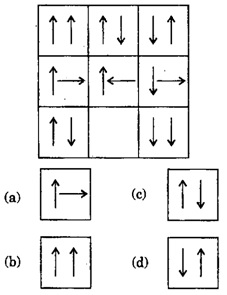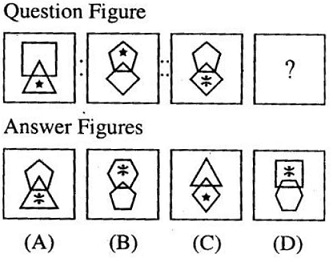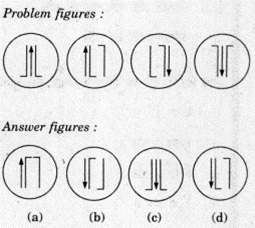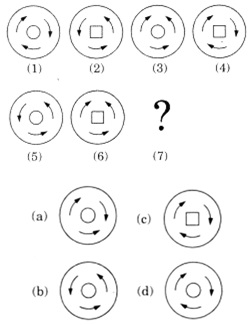# Non Verbal Reasoning Questions and Answers Part 2

5. Out of the given answer figures, which is the correct one to replace the empty boxa. B
b. D
c. A
d. C

Explanation:

Take first row. From 1st to 2nd figure, right side arrow inverts. From 2nd to 3rd figure, both arrow invert.
Same follows in 2nd row.
Same will follow in 3rd row. So, in 3rd row, from 1st to 2nd figure, just right arrow will invert.

6. Out of the given answer figures, which is the correct one to replace the question mark?a. D
b. C
c. A
d. B

Explanation:

A 4- sided figure has a 3 sided figure below it and 5 sided figure has 4 sided figure below it.
This rule is applicable only for figure B

7. Out of the given answer figures, which is the correct one to replace the question mark?a. B
b. D
c. C
d. A

Explanation:

Here in every figure there are three individual parts.
In first figure, second and third part (arrow and L) shifts left, and the first part shifts to the end and inverts.
In second diagram, same rule follows. Here the arrow is first part and arrow moves to the end and inverts.
Apply the same rule to get the answer.

8. What will be the seventh image in the sequence out of the given options a, b, c and d?a. c
b. b
c. d
d. a